## Proving equalities and identities

### Quick description

This page has links to other pages that discuss techniques for discovering and proving equalities and identities.

### General discussion

If one is asked to prove that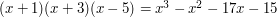, then the most obvious approach is to do a routine calculation – one simply expands out the brackets on the left-hand side and checks that the answer is the polynomial given on the right-hand side. However, in general there are many more interesting ways of proving equality of mathematical objects are equal. Indeed, even for this simple problem about polynomials one can use some theory to solve it, so we shall let it serve as our first non-obvious method for proving equality.

Technique 1: proving that two polynomials are the same by looking at the roots of their difference Brief summary (Two polynomials of degree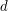are equal if they take the same value in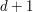different places. Proof: in this case their difference hasroots, but a non-zero polynomial of degreehas at mostroots. For the polynomial above, we can check that the right-hand side has zeros at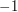,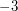and. Together with the observation that both polynomials take the value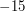when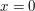(or alternatively, if one wants to use a tiny bit more theory, that they both have leading term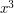), we find that they are equal. Although this method was not obviously the best one for this problem, sometimes it definitely is. See the article for some examples. )

Technique 2: double counting Brief summary ( One of the best ways of proving a non-obvious identity is to show that the two sides of the identity count or measure the same set in two different ways. For example, one could prove that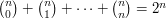by induction, but a much better proof is to observe that both sides are counting the number of subsets of an-element set. )

Technique 3: to prove that two real numbers are equal, prove that neither is greater than the other Brief summary ( To show that two real numbersand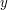are equal, it is often best to prove that it is not the case that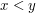and it is not the case that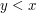. )

Technique 4: Axiomatics Brief summary ( How does one prove that the product ofandisin any field? The answer is that one uses the field axioms directly. Here is a proof. By the definition ofas an additive identity,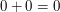. Therefore,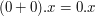. By the distributive law, the left-hand side is equal to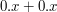, so we deduce that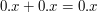. Adding the additive inverseof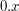to both sides we obtain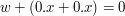. By the associativity of addition we can replace the left-hand side by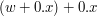, which equals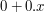. And by the definition of, this is, and the proof is complete. The article gives advice on how to come up with proofs of this kind. )

Technique 5: To prove that two objects are equal, show that in enough circumstances they behave in the same way Brief summary ( The polynomials method was an example of this. Another important one is that if two vectors have the same inner product with enough other vectors then they must be equal. An example will illustrate what this means. Suppose we have an-dimensional inner product space and an orthonormal basis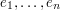, and would like to prove that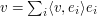for every. We can do so by taking the inner product ofwith an arbitrary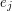. On the left-hand side we obtain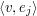, while on the right-hand side we obtain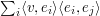. Since the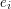are orthonormal, this also equals. )